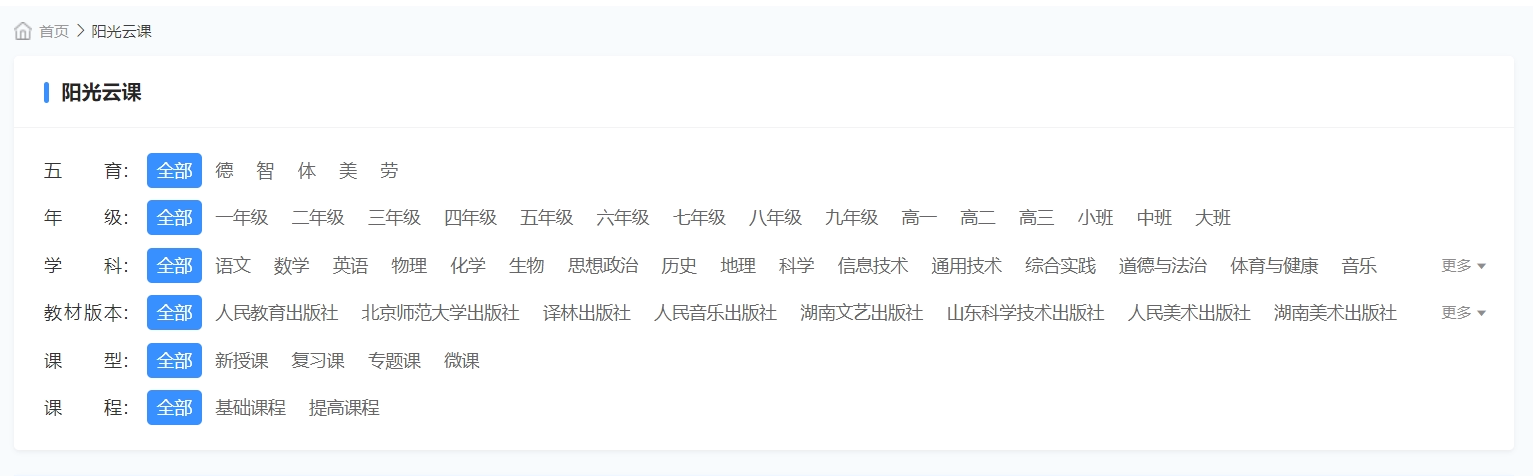• 累计撰写 45 篇文章
• 累计创建 40 个标签
• 累计收到 450 条评论

### 目 录CONTENT# 对象不支持"flat"属性或方法2021-02-09 / 0 评论 / 6 点赞 / 2,478 阅读 / 1,299 字 / 正在检测是否收录...广告

### 背景### 结果

😄以下代码引入即可解决浏览器不支持flat的问题。

if (!Array.prototype.flat) {
Array.prototype.flat = function (count) {
let c = count || 1;
let len = this.length;
let exe = [];
if (this.length == 0) return this;
while (c--) {
let _arr = [];
let flag = false;
if (exe.length == 0) {
flag = true;
for (let i = 0; i < len; i++) {
if (this[i] instanceof Array) {
exe.push(...this[i]);
} else {
exe.push(this[i]);
}
}
} else {
for (let i = 0; i < exe.length; i++) {
if (exe[i] instanceof Array) {
flag = true;
_arr.push(...exe[i]);
} else {
_arr.push(exe[i]);
}
}
exe = _arr;
}
if (!flag && c == Infinity) {
break;
}
}
return exe;
};
}


6
1.2.广告
• 6
1.2.